n queen topics
Home
Group actions
Search algorithm
Use symmetry, regular NQ problem
Evolution trees
Special properties
Necklaces
Conflicts
Conflict tables
Smallest solutions and greedy sequence
World records
Tables of counting results
Torus images for n=13
Other pages to the n queens problem
Bibliografy

Other themes
Primes from sums of factors
Primes from wrong binomic formula
Numbers by primes distribution

German

# Images for the normal n queens problem, n=8

Note: there are no torus solutions for n=8.

For the normal nQueens problem, there are 92 ways of placing the queens.

Ordering them by congruency in the square gives the decomposition

92 = 11 × 8 + 1 × 4

i.e. there are 12  solutions incongruent in the square (one of them having central symmetry).

Ordering them by congruency on the torus gives the decompositions

12 = 1 × 7 + 5 × 1,

i.e. 5 solutions are different also under torus shift, but the other 7 are equivalent;

92 = 1 × 56 + ( 4 × 8 + 1 × 4),

i.e. all solutions may be derived from six solutions on the torus.

These six solutions are given in the images below; they are moved to their lowest lexicografic representation.

## Explanation for the images

To understand the images, you may need some explanations: if two queens stand on the same diagonal, then there is a "conflict". These conflicts are shown as transparent lines, different conflicts using different colors. Of course, you should think the diagonals as loops on the torus, so there are two arcs between the two queens. The images contain only one of them.

For each conflict, there are "separation points" forming "separation areas" which consist or four rectangles; the images show these areas in the same color. A point (corner point of a field) is a separation point of a conflict if and only if that conflict disappears when you shift the origin to that point and view the arrangement as "normal queens".

If a point lies in the intersection of all separation areas, then I call it a separation point for the whole solution. These points are shown by little red circles.

## Table of imagesThe left solution may be described by "two big J"; the first "J" is in normal shape, formed by the queens at (0/0), (1/1), (2/3) and (3/6). The second J is reflected and shifted 4 rows up. This solution has two conflicts of length 1; it has symmetry for combination of reflection (at the vertical middle axis) and shift 4 rows. The solution on the right side may be described as "V made from knight lines, one leg shifted". The V is upside down, the first leg formed by the queens at (2/4), (1/2), (0/0) and (7/6), the second by (3/1), (4/7), (5/5) and (6/3). Note the 14 separation points! It has two conflicts of length 3, and has symmetry for combination of reflection (at the horizontal middle axis) and shift 4 columns. Note that these two solutions are similar, each an expansion by factor 3 of the other.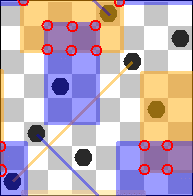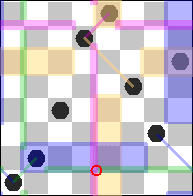These are solutions "Two small L" and "Two big L". The solution on the left side has four conflicts, as all following solutions; 2 conflicts are of length 1, 2 of length 2. For the solution on the right side, conflict lengths are 2 of 2, 2 of 3; both solutions have no symmetry. Again, these two solutions are similar, each an expansion by factor 3 of the other.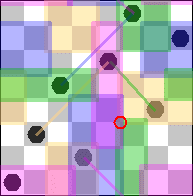The next solution is "Two knight lines, parallel". Conflict lengths are 1 of length 1, 3 of length 3; and it has central symmetry for (½/½) and (4½/4½).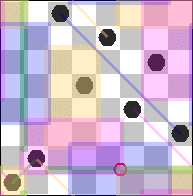It's expansion (not shown) also has four conflicts, 3 of length 1, 1 of length 3; but it has no separation point. The last solution may also be described as "Two small L"; the first formed of (6/2), (0/0) and (1/1), the second of (2/3), (4/5) and (3/6). It has again four conflicts, 2 of length 1, 2 of length 2, and has no symmetry.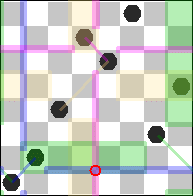Again, it's expansion has no separation point.

As you see, the idea of similarity gives a reduction from 6 to 4 "base" solutions. In the case of n=8, affine motions do not reduce the number of base solutions further.

Prepared by Matthias Engelhardt

last change: 2010-05-30